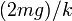# Block suspended vertically from spring

This article discusses a scenario/arrangement whose statics/dynamics/kinematics can be understood using the ideas of classical mechanics.
View other mechanics scenarios

In this article, we describe the following scenario. A block of mass$m$ is suspended vertically from a massless spring with spring constant$k$.

## Case that the spring is initially in its relaxed state

### Conclusion

In this case, the block and spring execute simple harmonic motion. Note that the mean position is not the relaxed state of the spring, because of the role that the gravitational force on the block plays in changing the equilibrium. Details about the oscillation are given below:

Quantity Value
Displacement of mean position of oscillations from usual relaxed state of spring$mg/k$
Amplitude of oscillation Also$(mg)/k$ (so the spring gets stretched by a maximum length of$(2mg)/k$ and thus oscillates between its relaxed position and a stretched position of$(2mg)/k$.
Angular frequency$\omega$ for oscillations$\sqrt{k/m}$
Frequency of oscillations (number of oscillations per second)$\frac{1}{2\pi} \sqrt{k/m}$
Time period of oscillation$2\pi \sqrt{m/k}$

## Other cases

In general, the displacement of mean position from the usual relaxed state of the spring remains$mg/k$ and the angular frequency, frequency of oscillations, and time period of oscillation remain the same. What can change is the amplitude of oscillations, which depends on the initial position and velocity of the system.